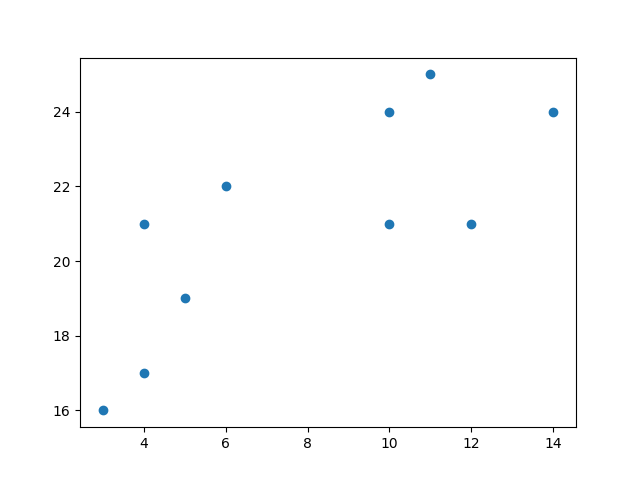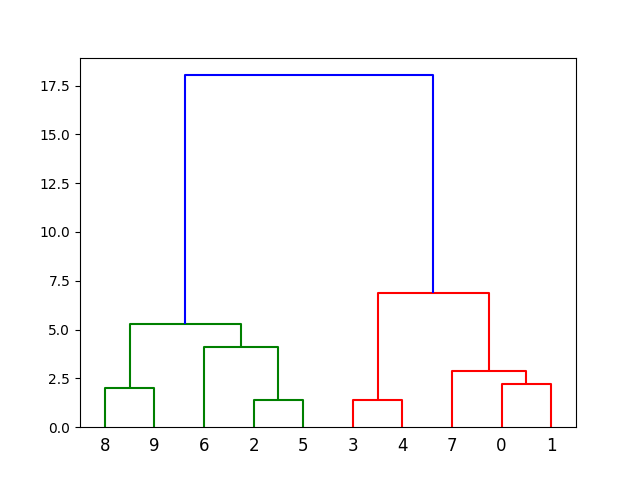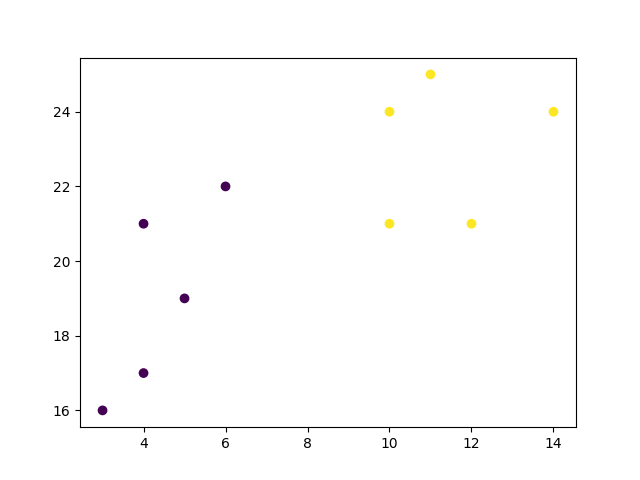## Python Tutorial

Python HOME Python Intro Python Get Started Python Syntax Python Comments Python Variables Python Data Types Python Numbers Python Casting Python Strings Python Booleans Python Operators Python Lists Python Tuples Python Sets Python Dictionaries Python If...Else Python While Loops Python For Loops Python Functions Python Lambda Python Arrays Python Classes/Objects Python Inheritance Python Iterators Python Scope Python Modules Python Dates Python Math Python JSON Python RegEx Python PIP Python Try...Except Python User Input Python String Formatting

## File Handling

Python File Handling Python Read Files Python Write/Create Files Python Delete Files

## Python Modules

NumPy Tutorial Pandas Tutorial SciPy Tutorial Django Tutorial

## Python Matplotlib

Matplotlib Intro Matplotlib Get Started Matplotlib Pyplot Matplotlib Plotting Matplotlib Markers Matplotlib Line Matplotlib Labels Matplotlib Grid Matplotlib Subplot Matplotlib Scatter Matplotlib Bars Matplotlib Histograms Matplotlib Pie Charts

## Machine Learning

Getting Started Mean Median Mode Standard Deviation Percentile Data Distribution Normal Data Distribution Scatter Plot Linear Regression Polynomial Regression Multiple Regression Scale Train/Test Decision Tree Confusion Matrix Hierarchical Clustering Logistic Regression Grid Search Categorical Data K-means Bootstrap Aggregation Cross Validation AUC - ROC Curve K-nearest neighbors

## Python MySQL

MySQL Get Started MySQL Create Database MySQL Create Table MySQL Insert MySQL Select MySQL Where MySQL Order By MySQL Delete MySQL Drop Table MySQL Update MySQL Limit MySQL Join

## Python MongoDB

MongoDB Get Started MongoDB Create Database MongoDB Create Collection MongoDB Insert MongoDB Find MongoDB Query MongoDB Sort MongoDB Delete MongoDB Drop Collection MongoDB Update MongoDB Limit

## Python Reference

Python Overview Python Built-in Functions Python String Methods Python List Methods Python Dictionary Methods Python Tuple Methods Python Set Methods Python File Methods Python Keywords Python Exceptions Python Glossary

## Module Reference

Random Module Requests Module Statistics Module Math Module cMath Module

## Python How To

Remove List Duplicates Reverse a String Add Two Numbers

## Python Examples

Python Examples Python Compiler Python Exercises Python Quiz Python Certificate

# Machine Learning - Hierarchical Clustering

On this page, W3schools.com collaborates with NYC Data Science Academy, to deliver digital training content to our students.

## Hierarchical Clustering

Hierarchical clustering is an unsupervised learning method for clustering data points. The algorithm builds clusters by measuring the dissimilarities between data. Unsupervised learning means that a model does not have to be trained, and we do not need a "target" variable. This method can be used on any data to visualize and interpret the relationship between individual data points.

Here we will use hierarchical clustering to group data points and visualize the clusters using both a dendrogram and scatter plot.

## How does it work?

We will use Agglomerative Clustering, a type of hierarchical clustering that follows a bottom up approach. We begin by treating each data point as its own cluster. Then, we join clusters together that have the shortest distance between them to create larger clusters. This step is repeated until one large cluster is formed containing all of the data points.

Hierarchical clustering requires us to decide on both a distance and linkage method. We will use euclidean distance and the Ward linkage method, which attempts to minimize the variance between clusters.

### Example

Start by visualizing some data points:

import numpy as np
import matplotlib.pyplot as plt

x = [4, 5, 10, 4, 3, 11, 14 , 6, 10, 12]
y = [21, 19, 24, 17, 16, 25, 24, 22, 21, 21]

plt.scatter(x, y)
plt.show()

### ResultRun example »

Now we compute the ward linkage using euclidean distance, and visualize it using a dendrogram:

### Example

import numpy as np
import matplotlib.pyplot as plt

x = [4, 5, 10, 4, 3, 11, 14 , 6, 10, 12]
y = [21, 19, 24, 17, 16, 25, 24, 22, 21, 21]

data = list(zip(x, y))

plt.show()

### ResultRun example »
Here, we do the same thing with Python's scikit-learn library. Then, visualize on a 2-dimensional plot:

### Example

import numpy as np
import matplotlib.pyplot as plt
from sklearn.cluster import AgglomerativeClustering

x = [4, 5, 10, 4, 3, 11, 14 , 6, 10, 12]
y = [21, 19, 24, 17, 16, 25, 24, 22, 21, 21]

data = list(zip(x, y))

labels = hierarchical_cluster.fit_predict(data)

plt.scatter(x, y, c=labels)
plt.show()

### ResultRun example »

## Example Explained

Import the modules you need.

```import numpy as np import matplotlib.pyplot as plt from scipy.cluster.hierarchy import dendrogram, linkage from sklearn.cluster import AgglomerativeClustering```

You can learn about the Matplotlib module in our "Matplotlib Tutorial.

You can learn about the SciPy module in our SciPy Tutorial.

NumPy is a library for working with arrays and matricies in Python, you can learn about the NumPy module in our NumPy Tutorial.

scikit-learn is a popular library for machine learning.

Create arrays that resemble two variables in a dataset. Note that while we only use two variables here, this method will work with any number of variables:

```x = [4, 5, 10, 4, 3, 11, 14 , 6, 10, 12] y = [21, 19, 24, 17, 16, 25, 24, 22, 21, 21] ```

Turn the data into a set of points:

```data = list(zip(x, y)) print(data) ```

Result:

```[(4, 21), (5, 19), (10, 24), (4, 17), (3, 16), (11, 25), (14, 24), (6, 22), (10, 21), (12, 21)] ```

Compute the linkage between all of the different points. Here we use a simple euclidean distance measure and Ward's linkage, which seeks to minimize the variance between clusters.

```linkage_data = linkage(data, method='ward', metric='euclidean') ```

Finally, plot the results in a dendrogram. This plot will show us the hierarchy of clusters from the bottom (individual points) to the top (a single cluster consisting of all data points).

`plt.show()` lets us visualize the dendrogram instead of just the raw linkage data.

```dendrogram(linkage_data) plt.show() ```

Result:The scikit-learn library allows us to use hierarchichal clustering in a different manner. First, we initialize the `AgglomerativeClustering` class with 2 clusters, using the same euclidean distance and Ward linkage.

```hierarchical_cluster = AgglomerativeClustering(n_clusters=2, affinity='euclidean', linkage='ward') ```

The `.fit_predict` method can be called on our data to compute the clusters using the defined parameters across our chosen number of clusters.

```labels = hierarchical_cluster.fit_predict(data) print(labels) ```

Result:

```[0 0 1 0 0 1 1 0 1 1] ```

Finally, if we plot the same data and color the points using the labels assigned to each index by the hierarchical clustering method, we can see the cluster each point was assigned to:

```plt.scatter(x, y, c=labels) plt.show() ```

Result: